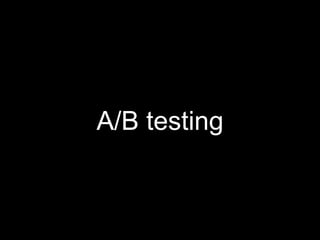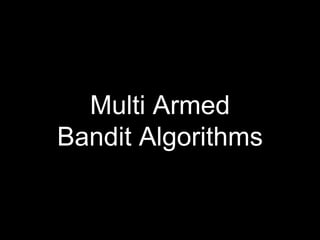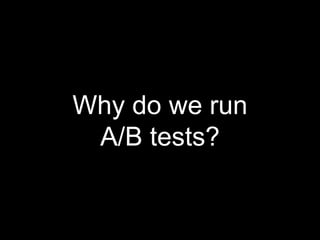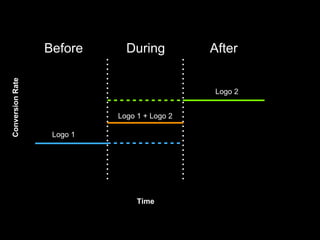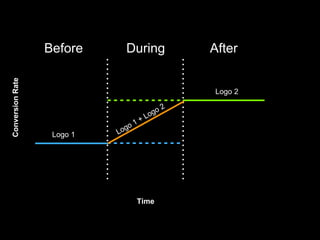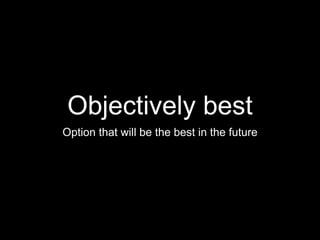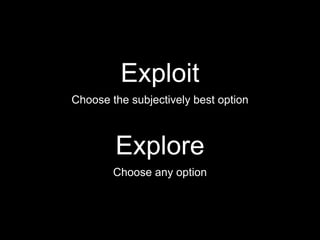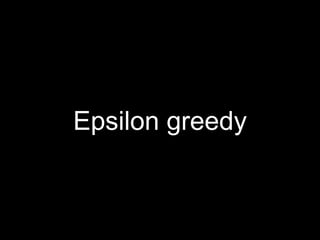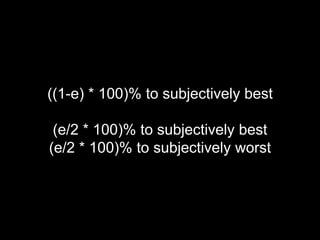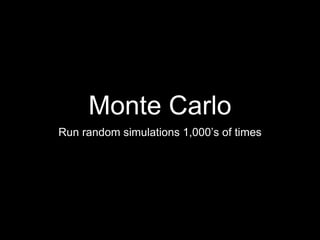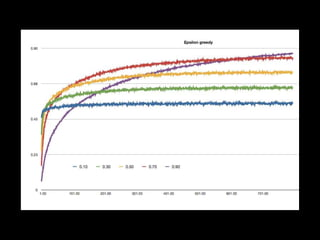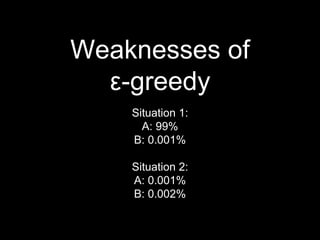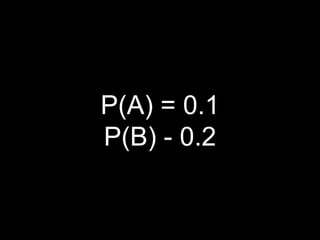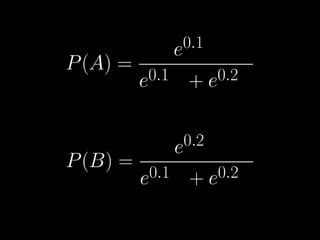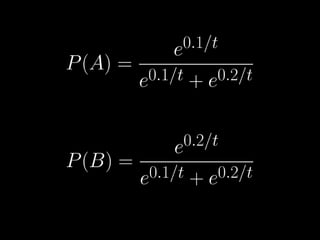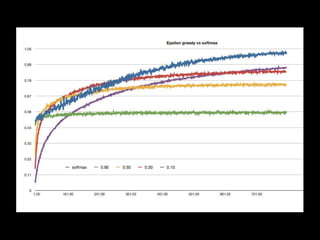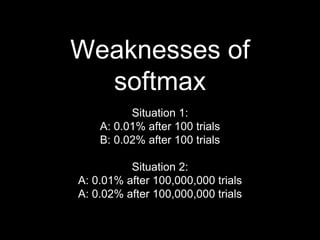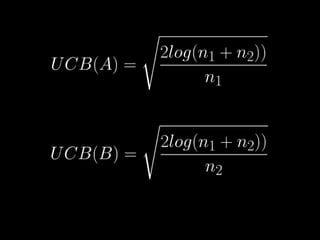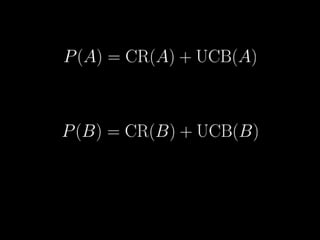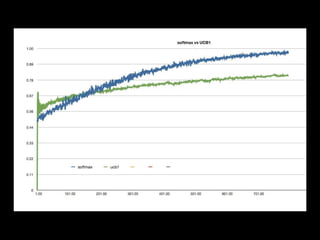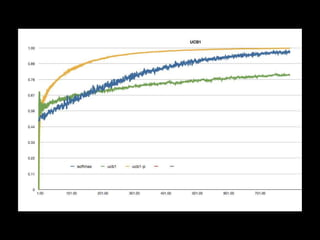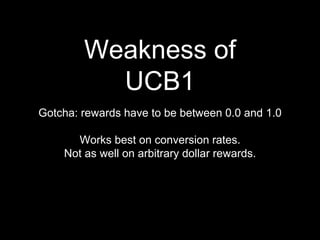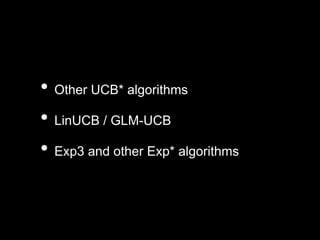1 of 41

### Website optimisation with Multi Armed Bandit algorithms

1. A/B testing
2. Multi Armed Bandit Algorithms
3. Why do we run A/B tests?
4. Before During After Logo 1 Logo 1 + Logo 2 Logo 2 ConversionRate Time
5. Before During After Logo 1 Logo 2 ConversionRate Time
6. Explore or exploit
7. Objectively best Option that will be the best in the future
8. Subjectively best Option that has been the best in the past
9. Explore Choose any option Exploit Choose the subjectively best option
10. Regret
11. Some classic Multi Armed Bandits...
12. Epsilon greedy
13. ((1-e) * 100)% to subjectively best (e/2 * 100)% to subjectively best (e/2 * 100)% to subjectively worst
14. Monte Carlo Run random simulations 1,000’s of times
15. Weaknesses of ε-greedy Situation 1: A: 99% B: 0.001% Situation 2: A: 0.001% B: 0.002%
16. Softmax
17. P(A) = 0.1 P(B) - 0.2
18. Weaknesses of softmax Situation 1: A: 0.01% after 100 trials B: 0.02% after 100 trials Situation 2: A: 0.01% after 100,000,000 trials A: 0.02% after 100,000,000 trials
19. UCB Upper Confidence Bound
20. Weakness of UCB1 Gotcha: rewards have to be between 0.0 and 1.0 Works best on conversion rates. Not as well on arbitrary dollar rewards.
22. • Other UCB* algorithms • LinUCB / GLM-UCB • Exp3 and other Exp* algorithms
23. Thanks!

### Editor's Notes

1. hello
2. Why run AB tests? because we might have some idea that something is better
3. The promise of MAB algorithms is that we can do something more like this. Ideally we want to be able to take advantage of what we learn as we go.
4. So there’s this dilemma between whether we exploit what we think is the best based on what we’ve seen or to explore other options to find out more about them.
5. MAB’s introduce this concept of regret. It’s how often did you have to try the objectively worst option in order to figure out the objectively best.
6. Lets look at a few classic MAB algorithms
7. Epsilon greedy works by alternating between exploration and exploitation. The name comes from the parameter epsilon that determines how much exploitation to do vs exploring.
8. So one of the weaknesses of epsilon greedy is that it doesn’t take into account of the proportional differences between variations.
9. Softmax attempts to address this by exploring options in proportion to how good they appear to be.
10. Lets say we’ve got two options and one is twice as good as the other.
11. So we could do a straight proportionality but instead softmax does this trick with exponentials so you can have rewards of arbitrary sizes but get back values between 0 and 1. The exponential thing kind of squishes it into a known range. You can even have negative rewards.
12. Softmax also has a concept of this “temperature”. Bigger temperature means more “energy”, more random.. closer to 50/50 A/B Lower number closer to 0 will explore the best option more in proportion to how good it is. Temp of 0 will be 100% exploitation.
13. So one of the weaknesses of softmax is it doesnt take into account how much you know about the diff options
14. Idea is to keep track of how much you know about each option gives you a measure of how confident you are about different options
15. There’s a whole family of UCB algorithms, but this is one called UCB1
16. So we take the observed conversion rate and add the confidence bound as a kind of bonus
17. So the main gotcha of UCB1 is that the payoff has to be between 0 and 1.
18. There are a bunch of variations of UCB algorithms. Also, contextual bandit algorithms that can take into account information about visitors. Exp3 algorithms are useful
19. Thanks to Lars. Also, thanks to John Myles White. This talk is based on a presentation of his.